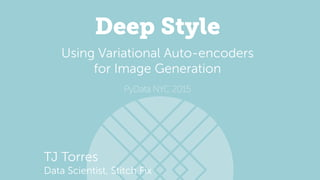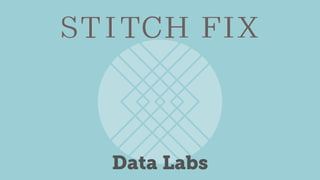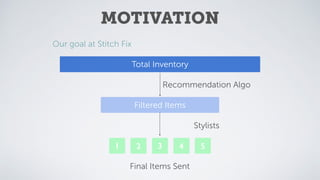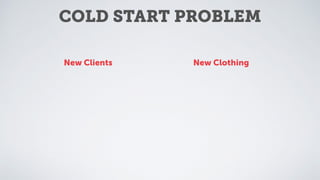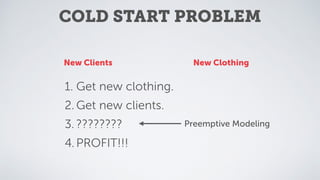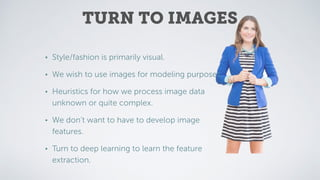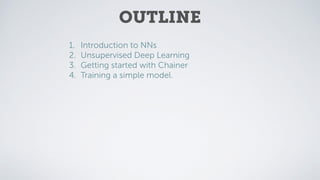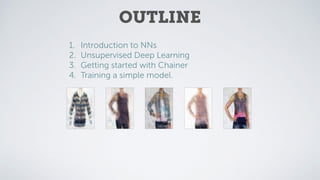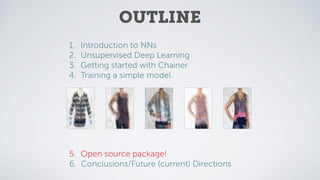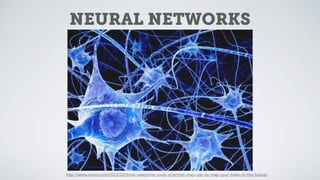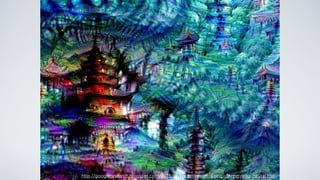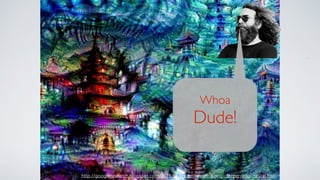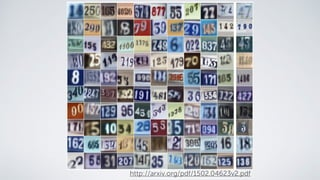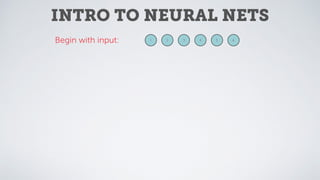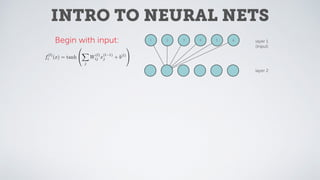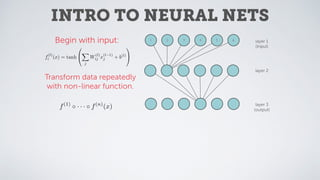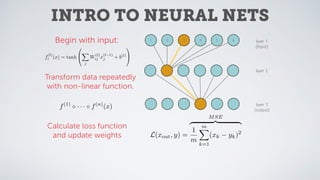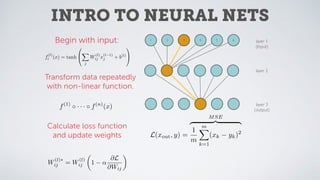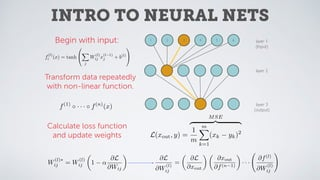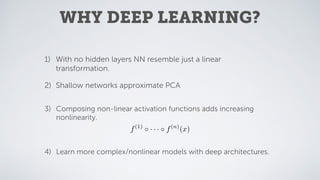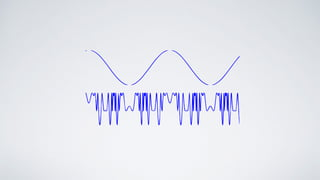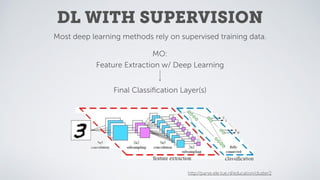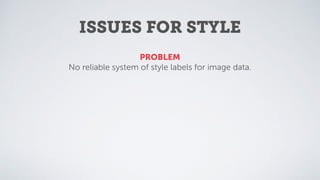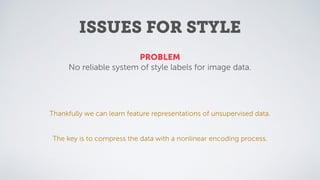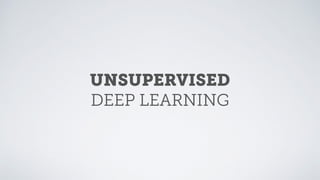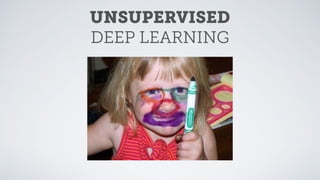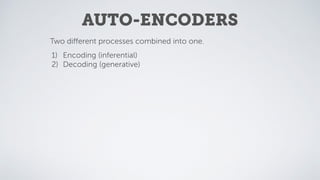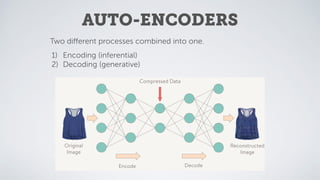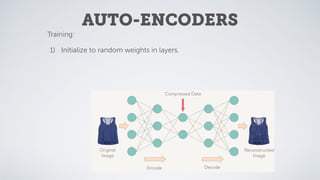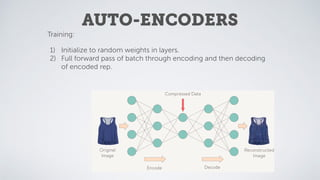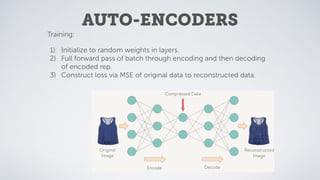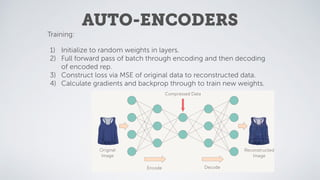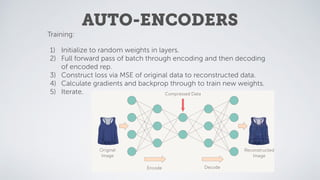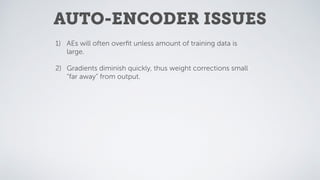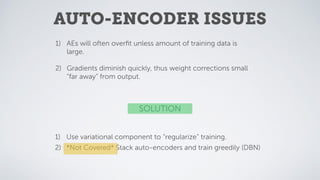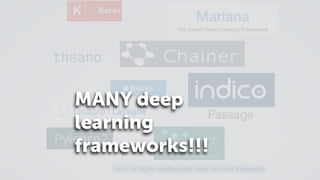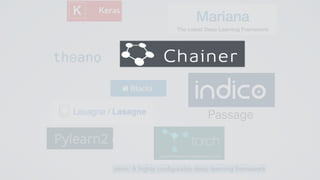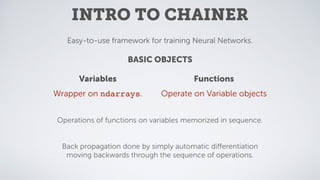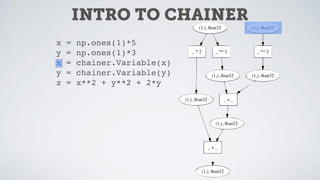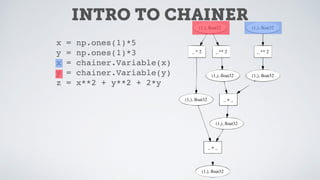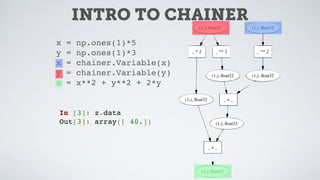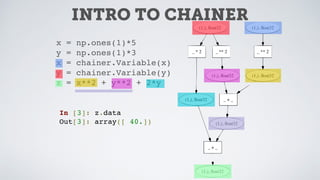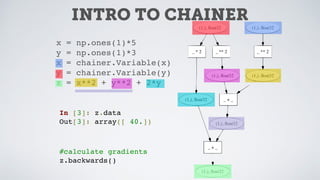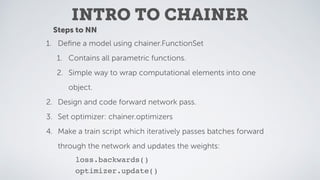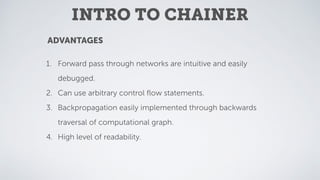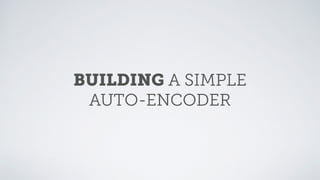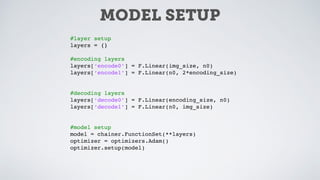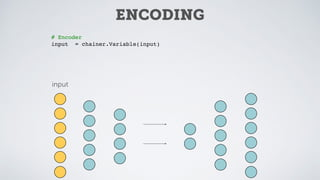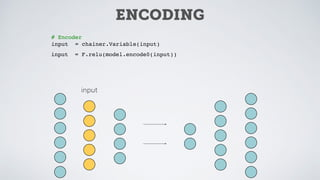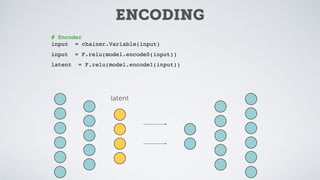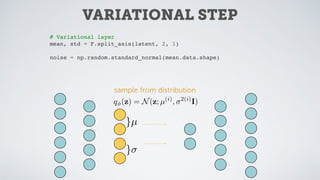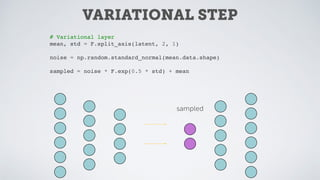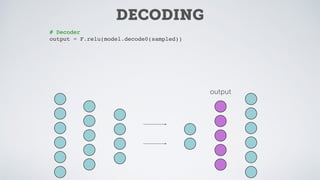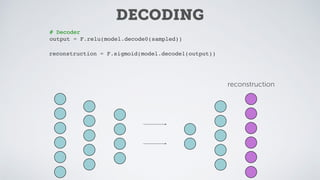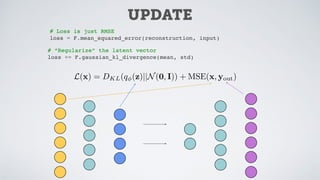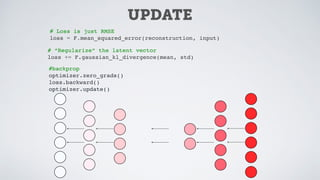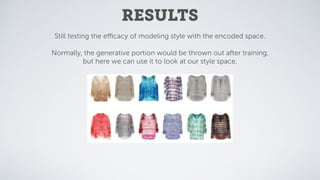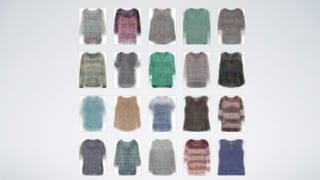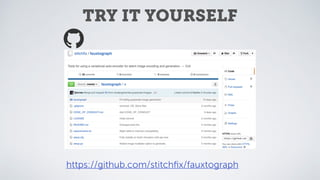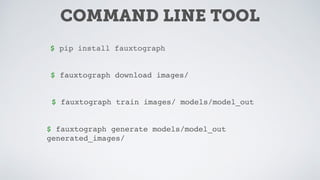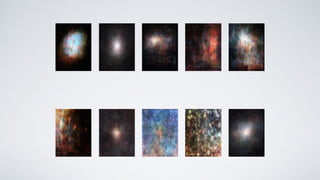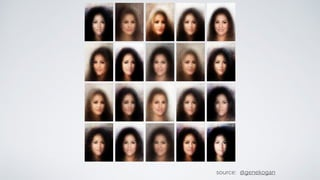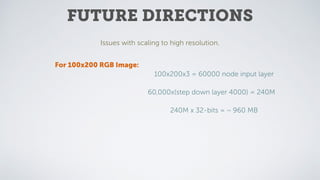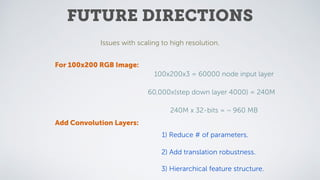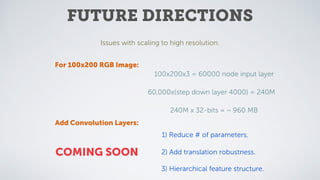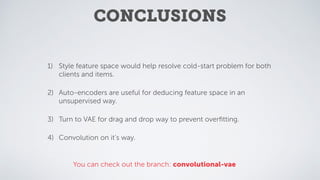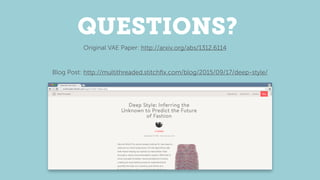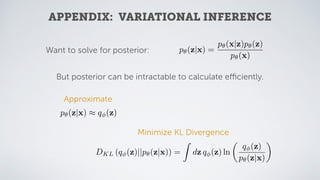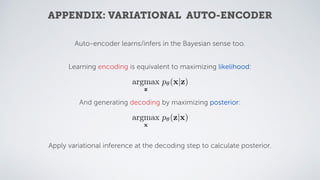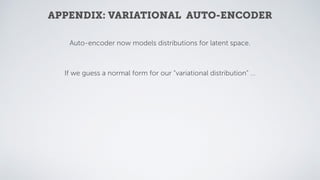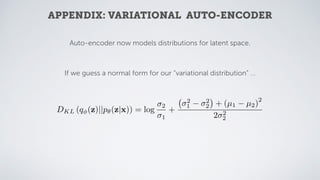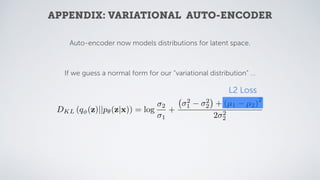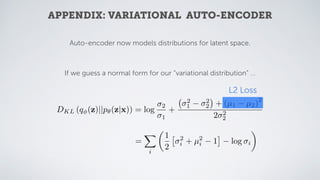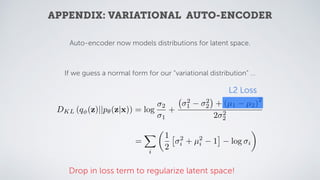1 de 80

### Deep Style: Using Variational Auto-encoders for Image Generation

1. Deep Style TJ Torres Data Scientist, Stitch Fix PyData NYC 2015 Using Variational Auto-encoders for Image Generation
2. Data Labs
3. Data Labs
4. Data Labs
5. Data Labs
6. MOTIVATION Our goal at Stitch Fix Total Inventory Recommendation Algo Stylists Filtered Items 1 2 3 4 5 Final Items Sent
7. COLD START PROBLEM New Clients New Clothing
8. New Clients New Clothing 1. Get new clothing. 2. Get new clients. 3. ???????? 4.PROFIT!!! COLD START PROBLEM
9. New Clients New Clothing 1. Get new clothing. 2. Get new clients. 3. ???????? 4.PROFIT!!! Preemptive Modeling COLD START PROBLEM
10. TURN TO IMAGES • Style/fashion is primarily visual. • We wish to use images for modeling purposes. • Heuristics for how we process image data unknown or quite complex. • We don’t want to have to develop image features. • Turn to deep learning to learn the feature extraction.
11. OUTLINE 1. Introduction to NNs 2. Unsupervised Deep Learning 3. Getting started with Chainer 4. Training a simple model.
12. 1. Introduction to NNs 2. Unsupervised Deep Learning 3. Getting started with Chainer 4. Training a simple model. OUTLINE
13. 1. Introduction to NNs 2. Unsupervised Deep Learning 3. Getting started with Chainer 4. Training a simple model. 5. Open source package! 6. Conclusions/Future (current) Directions OUTLINE
14. NEURAL NETWORKS http://www.wired.com/2013/02/three-awesome-tools-scientists-may-use-to-map-your-brain-in-the-future/
17. http://arxiv.org/pdf/1502.04623v2.pdf
18. Begin with input: INTRO TO NEURAL NETS 1 2 3 4 5 6
19. Begin with input: 1 2 3 4 layer 1 (Input) 5 6 layer 2 f (l) i (x) = tanh 0 @ X j W (l) ij x (l 1) j + b(l) 1 A INTRO TO NEURAL NETS
20. Begin with input: 1 2 3 4 layer 1 (Input) 5 6 layer 2 f (l) i (x) = tanh 0 @ X j W (l) ij x (l 1) j + b(l) 1 A layer 3 (output) Transform data repeatedly with non-linear function. f(1) · · · f(n) (x) INTRO TO NEURAL NETS
21. 1 2 3 4 layer 1 (Input) 5 6 layer 2 layer 3 (output) Calculate loss function and update weights f(1) · · · f(n) (x) L(xout, y) = MSE z }| { 1 m mX k=1 (xk yk)2 Begin with input: f (l) i (x) = tanh 0 @ X j W (l) ij x (l 1) j + b(l) 1 A Transform data repeatedly with non-linear function. INTRO TO NEURAL NETS
22. 1 2 3 4 layer 1 (Input) 5 6 layer 2 layer 3 (output) L(xout, y) = MSE z }| { 1 m mX k=1 (xk yk)2 W (l)⇤ ij = W (l) ij ✓ 1 ↵ @L @Wij ◆ Calculate loss function and update weights f(1) · · · f(n) (x) Begin with input: f (l) i (x) = tanh 0 @ X j W (l) ij x (l 1) j + b(l) 1 A Transform data repeatedly with non-linear function. INTRO TO NEURAL NETS
23. 1 2 3 4 layer 1 (Input) 5 6 layer 2 layer 3 (output) L(xout, y) = MSE z }| { 1 m mX k=1 (xk yk)2 W (l)⇤ ij = W (l) ij ✓ 1 ↵ @L @Wij ◆ @L @W (l) ij = ✓ @L @xout ◆ ✓ @xout @f(n 1) ◆ · · · @f(l) @W (l) ij ! Calculate loss function and update weights f(1) · · · f(n) (x) Begin with input: f (l) i (x) = tanh 0 @ X j W (l) ij x (l 1) j + b(l) 1 A Transform data repeatedly with non-linear function. INTRO TO NEURAL NETS
24. WHY DEEP LEARNING? 1) With no hidden layers NN resemble just a linear transformation. 2) Shallow networks approximate PCA 3) Composing non-linear activation functions adds increasing nonlinearity. f(1) · · · f(n) (x) 4) Learn more complex/nonlinear models with deep architectures.
25. DL WITH SUPERVISION Most deep learning methods rely on supervised training data. MO: Feature Extraction w/ Deep Learning Final Classiﬁcation Layer(s) http://parse.ele.tue.nl/education/cluster2
26. ISSUES FOR STYLE PROBLEM No reliable system of style labels for image data.
27. Thankfully we can learn feature representations of unsupervised data. The key is to compress the data with a nonlinear encoding process. PROBLEM No reliable system of style labels for image data. ISSUES FOR STYLE
28. UNSUPERVISED DEEP LEARNING
29. UNSUPERVISED DEEP LEARNING
30. AUTO-ENCODERS Two diﬀerent processes combined into one. 1) Encoding (inferential) 2) Decoding (generative)
31. Compressed Data Original Image Reconstructed Image Encode Decode Two diﬀerent processes combined into one. 1) Encoding (inferential) 2) Decoding (generative) AUTO-ENCODERS
32. Compressed Data Original Image Reconstructed Image Encode Decode AUTO-ENCODERS Training: 1) Initialize to random weights in layers. AUTO-ENCODERS
33. Compressed Data Original Image Reconstructed Image Encode Decode AUTO-ENCODERS Training: 1) Initialize to random weights in layers. 2) Full forward pass of batch through encoding and then decoding of encoded rep. AUTO-ENCODERS
34. Compressed Data Original Image Reconstructed Image Encode Decode AUTO-ENCODERS Training: 1) Initialize to random weights in layers. 2) Full forward pass of batch through encoding and then decoding of encoded rep. 3) Construct loss via MSE of original data to reconstructed data. AUTO-ENCODERS
35. Compressed Data Original Image Reconstructed Image Encode Decode AUTO-ENCODERS Training: 1) Initialize to random weights in layers. 2) Full forward pass of batch through encoding and then decoding of encoded rep. 3) Construct loss via MSE of original data to reconstructed data. 4) Calculate gradients and backprop through to train new weights. AUTO-ENCODERS
36. Compressed Data Original Image Reconstructed Image Encode Decode Training: 1) Initialize to random weights in layers. 2) Full forward pass of batch through encoding and then decoding of encoded rep. 3) Construct loss via MSE of original data to reconstructed data. 4) Calculate gradients and backprop through to train new weights. 5) Iterate. AUTO-ENCODERS
37. AUTO-ENCODER ISSUES 1) AEs will often overﬁt unless amount of training data is large. 2) Gradients diminish quickly, thus weight corrections small “far away” from output.
38. SOLUTION 1) Use variational component to “regularize” training. 2) *Not Covered* Stack auto-encoders and train greedily (DBN) 1) AEs will often overﬁt unless amount of training data is large. 2) Gradients diminish quickly, thus weight corrections small “far away” from output. AUTO-ENCODER ISSUES
39. Passage MANY deep learning frameworks!!!
40. Passage
41. Easy-to-use framework for training Neural Networks. BASIC OBJECTS Variables Functions Wrapper on ndarrays. Operate on Variable objects Operations of functions on variables memorized in sequence. Back propagation done by simply automatic diﬀerentiation moving backwards through the sequence of operations. INTRO TO CHAINER
42. x = np.ones(1)*5 y = np.ones(1)*3 x = chainer.Variable(x) y = chainer.Variable(y) z = x**2 + y**2 + 2*y INTRO TO CHAINER
43. x = np.ones(1)*5 y = np.ones(1)*3 x = chainer.Variable(x) y = chainer.Variable(y) z = x**2 + y**2 + 2*y INTRO TO CHAINER
44. x = np.ones(1)*5 y = np.ones(1)*3 x = chainer.Variable(x) y = chainer.Variable(y) z = x**2 + y**2 + 2*y In : z.data Out: array([ 40.]) INTRO TO CHAINER
45. x = np.ones(1)*5 y = np.ones(1)*3 x = chainer.Variable(x) y = chainer.Variable(y) z = x**2 + y**2 + 2*y In : z.data Out: array([ 40.]) INTRO TO CHAINER
46. x = np.ones(1)*5 y = np.ones(1)*3 x = chainer.Variable(x) y = chainer.Variable(y) z = x**2 + y**2 + 2*y In : z.data Out: array([ 40.]) #calculate gradients z.backwards() INTRO TO CHAINER
47. Steps to NN 1. Deﬁne a model using chainer.FunctionSet 1. Contains all parametric functions. 2. Simple way to wrap computational elements into one object. 2. Design and code forward network pass. 3. Set optimizer: chainer.optimizers 4. Make a train script which iteratively passes batches forward through the network and updates the weights: optimizer.update() loss.backwards() INTRO TO CHAINER
48. ADVANTAGES 1. Forward pass through networks are intuitive and easily debugged. 2. Can use arbitrary control ﬂow statements. 3. Backpropagation easily implemented through backwards traversal of computational graph. 4. High level of readability. INTRO TO CHAINER
49. BUILDING A SIMPLE AUTO-ENCODER
50. MODEL SETUP #layer setup layers = {} #encoding layers layers[‘encode0’] = F.Linear(img_size, n0) layers[‘encode1’] = F.Linear(n0, 2*encoding_size) #decoding layers layers[‘decode0’] = F.Linear(encoding_size, n0) layers[‘decode1’] = F.Linear(n0, img_size) #model setup model = chainer.FunctionSet(**layers) optimizer = optimizers.Adam() optimizer.setup(model)
51. ENCODING # Encoder input = chainer.Variable(input) input
52. # Encoder input = chainer.Variable(input) input = F.relu(model.encode0(input)) input ENCODING
53. # Encoder input = chainer.Variable(input) input = F.relu(model.encode0(input)) latent = F.relu(model.encode1(input)) latent ENCODING
54. VARIATIONAL STEP sample from distribution # Variational layer mean, std = F.split_axis(latent, 2, 1) noise = np.random.standard_normal(mean.data.shape) }µ } q (z) = N(z; µ(i) , 2(i) I)
55. VARIATIONAL STEP sampled # Variational layer mean, std = F.split_axis(latent, 2, 1) noise = np.random.standard_normal(mean.data.shape) sampled = noise * F.exp(0.5 * std) + mean
56. DECODING # Decoder output = F.relu(model.decode0(sampled)) output
57. DECODING # Decoder output = F.relu(model.decode0(sampled)) reconstruction = F.sigmoid(model.decode1(output)) reconstruction
58. UPDATE # Loss is just RMSE loss = F.mean_squared_error(reconstruction, input) # “Regularize” the latent vector loss += F.gaussian_kl_divergence(mean, std) L(x) = DKL(q (z)||N(0, I)) + MSE(x, yout)
59. UPDATE # Loss is just RMSE loss = F.mean_squared_error(reconstruction, input) # “Regularize” the latent vector loss += F.gaussian_kl_divergence(mean, std) #backprop optimizer.zero_grads() loss.backward() optimizer.update()
60. AFTER TRAINING
61. RESULTS Still testing the eﬃcacy of modeling style with the encoded space. Normally, the generative portion would be thrown out after training, but here we can use it to look at our style space.
62. TRY IT YOURSELF https://github.com/stitchﬁx/fauxtograph
63. COMMAND LINE TOOL \$ pip install fauxtograph \$ fauxtograph download images/ \$ fauxtograph train images/ models/model_out \$ fauxtograph generate models/model_out generated_images/
64. source: @genekogan
65. FUTURE DIRECTIONS Issues with scaling to high resolution.
66. For 100x200 RGB Image: 100x200x3 = 60000 node input layer 60,000x(step down layer 4000) = 240M 240M x 32-bits = ~ 960 MB FUTURE DIRECTIONS Issues with scaling to high resolution.
67. Add Convolution Layers: 1) Reduce # of parameters. 2) Add translation robustness. 3) Hierarchical feature structure. FUTURE DIRECTIONS For 100x200 RGB Image: 100x200x3 = 60000 node input layer 60,000x(step down layer 4000) = 240M 240M x 32-bits = ~ 960 MB Issues with scaling to high resolution.
68. Add Convolution Layers: 1) Reduce # of parameters. 2) Add translation robustness. 3) Hierarchical feature structure. FUTURE DIRECTIONS For 100x200 RGB Image: 100x200x3 = 60000 node input layer 60,000x(step down layer 4000) = 240M 240M x 32-bits = ~ 960 MB Issues with scaling to high resolution. COMING SOON
69. CONCLUSIONS 1) Style feature space would help resolve cold-start problem for both clients and items. 2) Auto-encoders are useful for deducing feature space in an unsupervised way. 3) Turn to VAE for drag and drop way to prevent overﬁtting. 4) Convolution on it’s way. You can check out the branch: convolutional-vae
70. QUESTIONS? Original VAE Paper: http://arxiv.org/abs/1312.6114 Blog Post: http://multithreaded.stitchﬁx.com/blog/2015/09/17/deep-style/
71. APPENDIX: VARIATIONAL INFERENCE Want to solve for posterior: p✓(z|x) = p✓(x|z)p✓(z) p✓(x) But posterior can be intractable to calculate eﬃciently. Approximate p✓(z|x) ⇡ q (z) Minimize KL Divergence DKL (q (z)||p✓(z|x)) = Z dz q (z) ln ✓ q (z) p✓(z|x) ◆
72. APPENDIX: VARIATIONAL AUTO-ENCODER Auto-encoder learns/infers in the Bayesian sense too. Learning encoding is equivalent to maximizing likelihood: argmax z p✓(x|z) And generating decoding by maximizing posterior: argmax x p✓(z|x) Apply variational inference at the decoding step to calculate posterior.
73. Auto-encoder now models distributions for latent space. If we guess a normal form for our “variational distribution” … APPENDIX: VARIATIONAL AUTO-ENCODER
74. DKL (q (z)||p✓(z|x)) = log 2 1 + 2 1 2 2 + (µ1 µ2) 2 2 2 2 Auto-encoder now models distributions for latent space. If we guess a normal form for our “variational distribution” … APPENDIX: VARIATIONAL AUTO-ENCODER
75. DKL (q (z)||p✓(z|x)) = log 2 1 + 2 1 2 2 + (µ1 µ2) 2 2 2 2 L2 Loss Auto-encoder now models distributions for latent space. If we guess a normal form for our “variational distribution” … APPENDIX: VARIATIONAL AUTO-ENCODER
76. DKL (q (z)||p✓(z|x)) = log 2 1 + 2 1 2 2 + (µ1 µ2) 2 2 2 2 L2 Loss = X i ✓ 1 2 ⇥ 2 i + µ2 i 1 ⇤ log i ◆ Auto-encoder now models distributions for latent space. If we guess a normal form for our “variational distribution” … APPENDIX: VARIATIONAL AUTO-ENCODER
77. DKL (q (z)||p✓(z|x)) = log 2 1 + 2 1 2 2 + (µ1 µ2) 2 2 2 2 L2 Loss = X i ✓ 1 2 ⇥ 2 i + µ2 i 1 ⇤ log i ◆ Drop in loss term to regularize latent space! Auto-encoder now models distributions for latent space. If we guess a normal form for our “variational distribution” … APPENDIX: VARIATIONAL AUTO-ENCODER